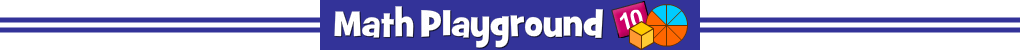Find the prime factors of each number.
Use prime factorization to find the GCF and LCM.
Factor Trees - Learning Connections
Essential Skills
Number Properties - identify prime factors

Common Core Connection for Grades 4, 5, 6
Determine whether a given whole number in the range 1-100 is prime or composite.
Find common factors and multiples.
Find the greatest common factor of two whole numbers less than or equal to 100.
Find the least common multiple of two whole numbers less than or equal to 12.
More Math Games to Play